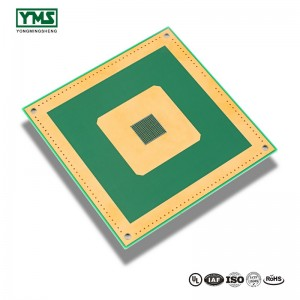Welcome to our website.

## Ounce is a unit of weight. Why is it used to indicate thickness in PCB?

The first thing to note is that the ounce is itself a unit of weight.

Ounces and grams (g) are converted to 1OZ ≈28.35g.

In the PCB industry, 1OZ means the thickness of 1OZ of copper spread evenly over an area of 1 square foot (FT2).

It is expressed by weight per unit area as the average thickness of copper foil. Using the formula, 1OZ=28.35g/ FT2 (FT2 =0.09290304 m2).

Specifically, the conversion method of length and thickness is as follows:

First, we know that the density constant of copper and the relevant unit conversion formula are as follows:

The density of copper p =8.9g/cm3

1 cm (cm) =10 mm (mm); 1 mm (mm) =1000 micron (um)

1 mil material 25.4 um

1 FT2 material 929.0304 cm2

1 mil material 25.4 um

According to the mass calculation formula m = rho ×V (volume) = rho ×S (area) ×t (thickness), know the weight of copper foil divided by the density of copper and the area is the thickness of copper foil.

As previously known, 1OZ=t×929.0304cm2×8.9g/ cm3=28.35g

So, t = 28.35/ 929.0304/ 8.9 cm ≈ 0.0034287 cm = 34.287 um material 34.287/ 25.4 mil≈ 1.35 mil

Therefore, the thickness of 1OZ copper foil is about 35um or 1.35mil.

Copper thickness 1.OZ(0.035mm) copper thickness 1.5OZ(0.05mm) copper thickness 2.OZ(0.07mm)

### 1. PCB line width and current relationship

First, calculate the cross-sectional area of Track, most of the copper foil thickness of PCB is 35um (if you are not sure, you can ask the PCB manufacturer), which is the cross-sectional area multiplied by the online width, and pay attention to convert it into square millimeters.

There is an empirical current density of 15~25 amperes per square millimeter. Call it the cross section and you get the flow capacity.

### 2. Data:

The calculation of PCB current carrying capacity is always lack of authoritative technical methods and formulas. Experienced CAD engineers can make accurate judgments based on their personal experience. But for CAD novice, can not be said to encounter a difficult problem.

The current carrying capacity of PCB depends on the following factors: line width, line thickness (copper foil thickness), allowed temperature rise. As we all know, the wider the PCB, the greater the current carrying capacity.

Here, please tell me: assuming that under the same conditions, a 10MIL wire can withstand 1A, how much current can a 50MIL wire withstand? Is it 5A? The answer, of course, is no.

Please refer to the following data provided by international authorities:

The units of wire width are: 1 oz. Copper =35 micron thick, 2 oz.=70 micron thick, 1 oz =0.035mm 1mil.=10-3 Inch.

The voltage drop due to wire resistance due to wire length must also be considered in the experiment. The process only welds the tin to increase the current capacity, but it is difficult to control the volume of tin.

1OZ copper, 1mm wide, generally used as A 1-3 A galvanometer, depending on your line length and requirements for pressure drop.

The maximum current value should be the maximum allowable value under the temperature rise limit, the fuse value is the temperature rise to the melting point of the copper. Eg. 50mil 1oz temperature rise of 1060 degrees (that is, the melting point of copper), the current is 22.8A.

## You May Like:

### pcb copper### metal core pcb 1Layer

Post time: Sep-07-2019